# Electronics and Communication Engineering - Exam Questions Papers

41.
The Z-inverse of the given Z-transform is __________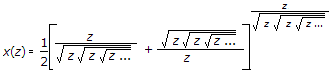unit step
unit ramp
unit impulse
unit parabola
Explanation:value of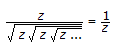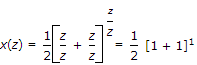x(n) = d(n) impulse response.

42.
Find the Fourier transform of the half cosine pulse as shown below :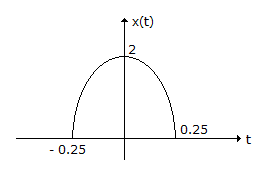0.5 {sin c [0.25(f - 1)] + sin c [2.5(f + 1)]}
0.5 {sin c [0.5(f - 1)] + sin c [0.5(f + 1)]}
0.25 {sin c [0.25(f - 1)] + sin c [0.25 (f - 1)]}
0.25 {sin c [0.5(f - 1)] + sin c [0.5(f - 1)]}
Explanation:

The given signal can be expressed as multiplication of x1(t) and x2(t) as shown below.

where A = 2, T/2 = 0.25 => T = 0.5

x(t) = x1(t) x x2(t)

=> X(f) = X1(f) * X2(f)

Now X1(f) =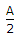[δ(f - f0) + δ(f + f0)]

=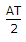[sin c [T(f - f0)] + sin c[T(f + f0)]]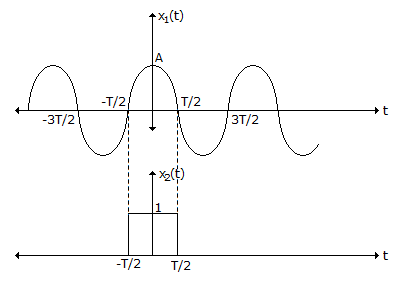Now, A = 2, T = 0.5 and f0 =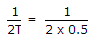= 1

=> X(f) = 0.5[sin c (0.5(f - 1)) + sin c (0.5(f + 1))].

43.
The q-v relation of a capacitor is v = 1 + q + qx. Find the amount of energy required to charge this capacitor from q(t0) = 0 to q(t) = 5 C.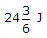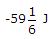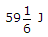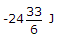Explanation: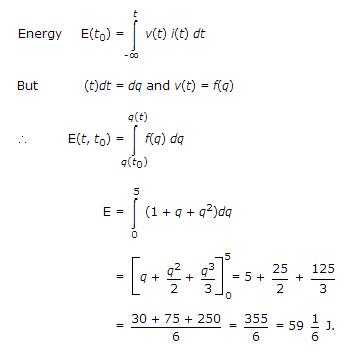44.
V-I relationship of a network N is given by, V = 8 - 4I.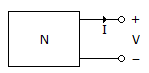The equivalent circuit to the network N is shown below
The values of IN and RN are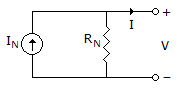0, 2
0, 4
2, 4
None of these
Explanation:

Given equivalent circuit is Norton's equivalent circuit

V = 8 - 4I

Put V = 0I = IN = 2 A

Put I = 0 V = VTH = 8 V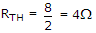.

45.
If the open-loop transfer function is a ratio of a numerator polynomial of degree 'm' and a denominator polynomial of degree 'n', then the integer (n - m) represents the number of:
breakaway points
unstable poles
separate root loci
asymptotes